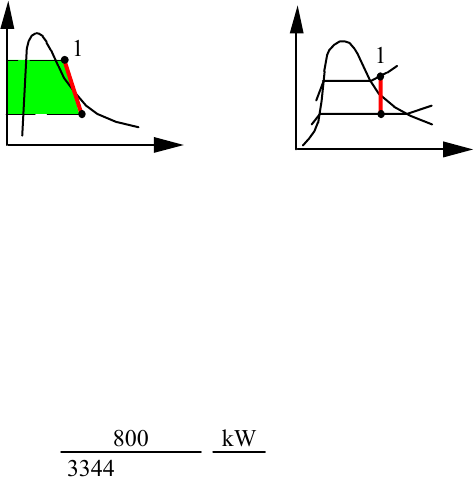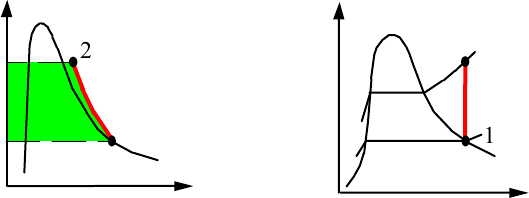Textbook Notes (280,000)
US (110,000)
LSU (20,000)
ME (300)
ME 3333 (50)
All (50)
Chapter

# Homework 11 SolutionsExam

Department
Mechanical Engineering
Course Code
ME 3333
Professor
All

This preview shows page 1. to view the full 5 pages of the document.Borgnakke and Sonntag
9.17
Steam enters a turbine at 3 MPa, 450°C, expands in a reversible adiabatic process
and exhausts at 10 kPa. Changes in kinetic and potential energies between the
inlet and the exit of the turbine are small. The power output of the turbine is 800
kW. What is the mass flow rate of steam through the turbine?
Solution:
. = 0.
Continuity Eq.6.11: m
.
i = m
.
e = m
.,
Energy Eq.6.12: m
.hi = m
.he + W
.
T,
Entropy Eq.9.8: m
.si + 0/ = m
.se ( Reversible S
.
gen = 0 )
Explanation for the
work term is in Sect.
9.3, Eq.9.14 2
1
P
v
T
s
1
2
Inlet state: Table B.1.3 hi = 3344 kJ/kg, si = 7.0833 kJ/kg K
Exit state: Pe , se = si Table B.1.2 saturated as se < sg
x
e = (7.0833 - 0.6492)/7.501 = 0.8578,
h
e = 191.81 + 0.8578 × 2392.82 = 2244.4 kJ/kg
m
. = W
.
T/wT = W
.
T/(hi - he) = 800
3344 - 2244.4 kW
kJ/kg = 0.728 kg/s
Excerpts from this work may be reproduced by instructors for distribution on a not-for-profit basis for
testing or instructional purposes only to students enrolled in courses for which this textbook has been
adopted. Any other reproduction or translation of this work beyond that permitted by Sections 107 or 108
of the 1976 United States Copyright Act without the permission of the copyright owner is unlawful.

Unlock to view full version

Only page 1 are available for preview. Some parts have been intentionally blurred.Borgnakke and Sonntag
9.18
200 kPa and has an exit presure of 800 kPa. Neglect kinetic energies and find the
exit temperature and the minimum power needed to drive the unit.
Solution:
. = 0.
Continuity Eq.6.11: m
.
i = m
.
e = m
.,
Energy Eq.6.12: m
.hi = m
.he + W
.
C,
Entropy Eq.9.8: m
.si + 0/ = m
.se ( Reversible S
.
gen = 0 )
Inlet state: B 4.2.: hi = 264.27 kJ/kg, si = 1.1192 kJ/kg K
Exit state: Pe , se = si Table B.4.2 he = 302.65 kJ/kg, Te 22.7°C
–wc = he - hi = 302.65 – 264.27 = 38.38 kJ/kg
– W
.
c = Power In = –wcm
. = 38.38 × 0.05 = 1.92 kW
Explanation for the
work term is in Sect.
9.3, Eq.9.18
2
1
P
v
T
s
1
2
Excerpts from this work may be reproduced by instructors for distribution on a not-for-profit basis for
testing or instructional purposes only to students enrolled in courses for which this textbook has been
adopted. Any other reproduction or translation of this work beyond that permitted by Sections 107 or 108
of the 1976 United States Copyright Act without the permission of the copyright owner is unlawful.